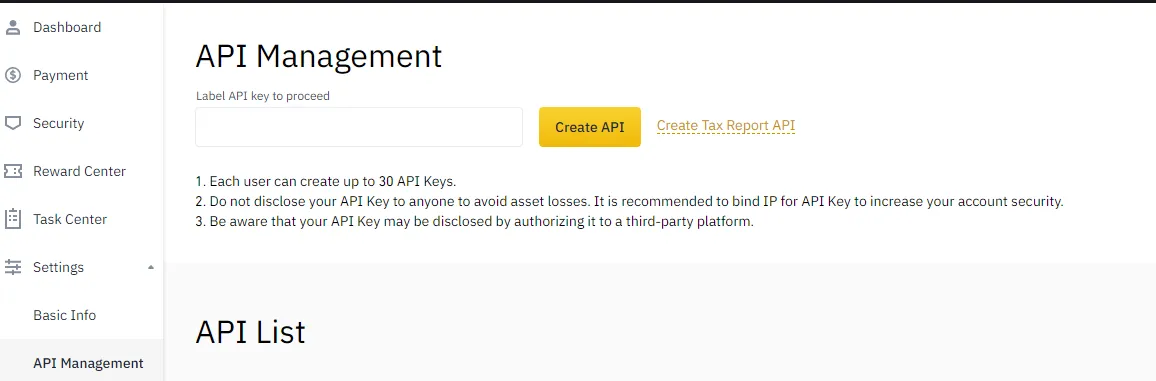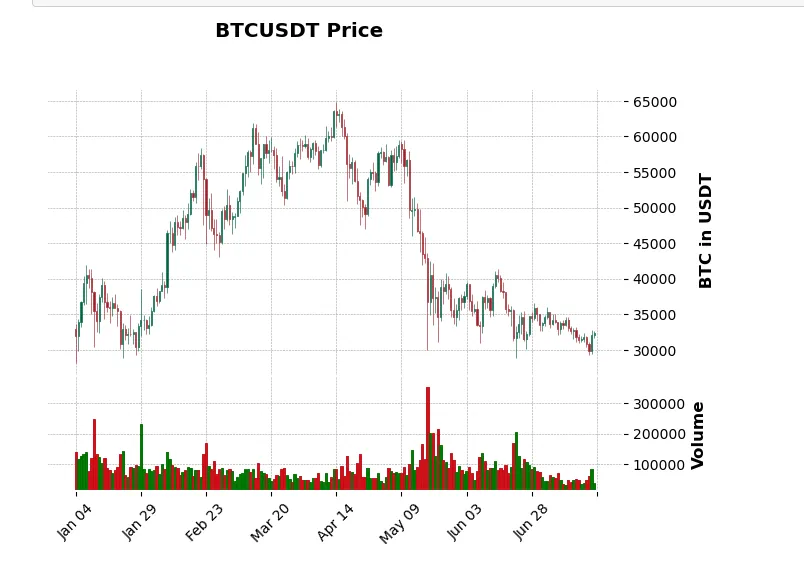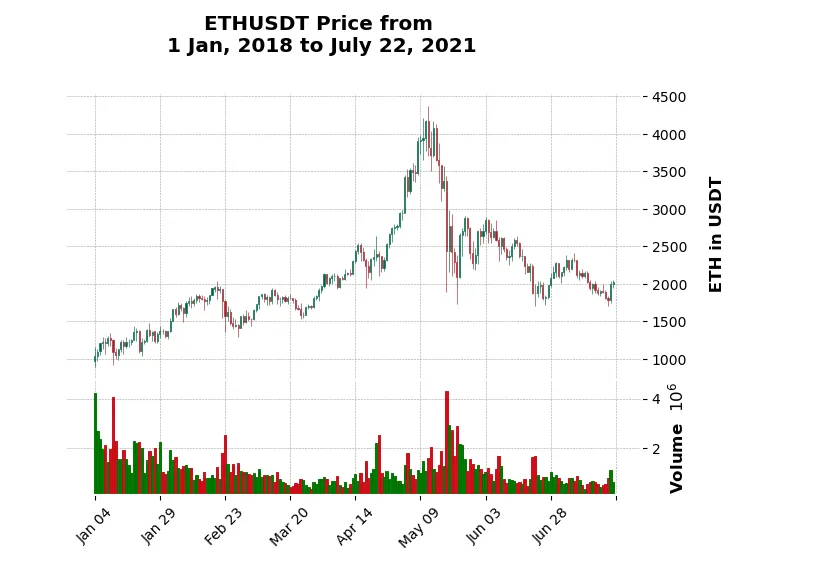# Python With Binance Crypto Price Data

Hi everyone. In this programming post, I cover the topic of using Python with Binance crypto price data. This is from a personal project where I play around with Python and Binance price data to produce candlestick price charts. The main reference that I use is this Youtube video from Nicholas Renotte.

The link to my jupyterNotebook project is here.Pixabay Image Source

## Topics

• Setup of Binance API
• Some Functions
• Obtaining Historical Data of Bitcoin/Tether (BTC/USDT)
• Preprocessing Data
• Candlestick Price Plots On Binance Crypto Data
• Creating A Function For Producing Candlestick Price Charts Given Inputs

## Setup of Binance API

Setting up the connection from Python (jupyterNotebook) to the Binance API is not too difficult. Go to your Binance account and obtain an API key there. This is done through the Profile picture icon then API Management.For installation, you need the `python-binance` package installed. You can use `pip install python-binance`. The following code uses the API key and the secret key obtained from Binance and inputs them into Client.

``````
import pandas as pd

client = Client(api_key, secret_key)

``````Pixabay Image Source

## Some Functions

With the Client, you can obtain data from Binance. I test out a few functions.

``````
# Obtain tickers:

tickers = client.get_all_tickers()

# First ten tickers
tickers[0:10]

[{'symbol': 'ETHBTC', 'price': '0.06240000'},
{'symbol': 'LTCBTC', 'price': '0.00374400'},
{'symbol': 'BNBBTC', 'price': '0.00912300'},
{'symbol': 'NEOBTC', 'price': '0.00090000'},
{'symbol': 'QTUMETH', 'price': '0.00277900'},
{'symbol': 'EOSETH', 'price': '0.00175500'},
{'symbol': 'SNTETH', 'price': '0.00003343'},
{'symbol': 'BNTETH', 'price': '0.00142400'},
{'symbol': 'BCCBTC', 'price': '0.07908100'},
{'symbol': 'GASBTC', 'price': '0.00018060'}]

``````

With list comprehension, the crypto pairs alone can be obtained:

``````# Obtain tickers pairs with list comprehension:

ticker_pairs = [x['symbol'] for x in tickers]

ticker_pairs[0:10]

['ETHBTC',
'LTCBTC',
'BNBBTC',
'NEOBTC',
'QTUMETH',
'EOSETH',
'SNTETH',
'BNTETH',
'BCCBTC',
'GASBTC']
``````

A list comprehension with an if statement can be used for obtaining crypto pairs where one of the cryptos is Tether (USDT):

``````# Obtain ticker pairs in USDT (Tether) as Tether is common in pairs for transactions:

usdt_pairs = [x['symbol'] for x in tickers if 'USDT' in x['symbol']]

usdt_pairs[0:10]

['BTCUSDT',
'ETHUSDT',
'BNBUSDT',
'BCCUSDT',
'NEOUSDT',
'LTCUSDT',
'QTUMUSDT',
'XRPUSDT',
'EOSUSDT']
``````Pixabay Image Source

## Obtaining Historical Data of Bitcoin/Tether (BTC/USDT)

As an example, I obtain histoical price data of the BTC/USDT crypto pair.

``````# Crypto trading pair:

base_cur = 'BTC'
quote_cur = 'USDT'

user_pair = base_cur + quote_cur

# Obtain 1 day timeframe data for BTCUSDT trading pair:

klines = client.get_historical_klines(user_pair , Client.KLINE_INTERVAL_1DAY, "1 Dec, 2017", "July 22, 2021")

# Sample output
# It is a list of lists.
# Open time, Open, High, Low, Close, Volume, Close Time, Quote Asset Volume, Num Trades, Taker buy base asset vol,
# Taker buy quote asset vol, Ignore.

klines[0:3]

[[1512086400000,
'9837.00000000',
'10898.00000000',
'9380.00000000',
'10782.99000000',
'6134.92363300',
1512172799999,
'62260697.58291551',
32375,
'3269.86858800',
'33193672.16592109',
'35258.70264511'],
[1512172800000,
'10775.04000000',
'11190.00000000',
'10620.00000000',
'10890.01000000',
'4765.43975700',
1512259199999,
'52046689.84006951',
29694,
'2390.19427500',
'26111051.97904352',
'35491.76836387'],
[1512259200000,
'10902.69000000',
'11825.00000000',
'10500.00000000',
'11165.41000000',
'5346.63652400',
1512345599999,
'60350708.29332783',
39335,
'2574.24618700',
'29090180.16290429',
'36967.53447487']]

``````

The function for obtaining historical price data is `client.get_historical_klines(<crypto_pair> , <timeframe>, <start_date>, <end_date>)`.

With the timeframe, it is not as simple as `1day, 1min or 1 month`. The `KLINE_INTERVAL` part is needed. Here is the documentation link
which has more interval examples.Pixabay Image Source

## Preprocessing Data

The historical price data for BTCUSDT is then put into a pandas dataframe. Columns are renamed too.

``````# Convert klines historical data into Pandas dataframe:

btc_usdt_df = pd.DataFrame(klines)

# Name colums:

col_names = ['Open Time', 'Open', 'High', 'Low', 'Close', 'Volume', 'Close Time', 'Quote Asset Volume',                  'Num Trades', 'Taker buy base asset vol', 'Taker buy quote asset vol', 'Ignore']

btc_usdt_df.columns = col_names

``````

Times are converted from UNIX format into datetime and the rest of the columns are converted into numeric columns.

``````# UNIX to datetime (divide by 1000):

btc_usdt_df['Open Time'] = pd.to_datetime(btc_usdt_df['Open Time']/1000, unit = 's')
btc_usdt_df['Close Time'] = pd.to_datetime(btc_usdt_df['Close Time']/1000, unit = 's')

numeric_cols = ['Open', 'High', 'Low', 'Close', 'Volume', 'Quote Asset Volume', 'Num Trades',

btc_usdt_df[numeric_cols] = btc_usdt_df[numeric_cols].apply(pd.to_numeric, axis = 1)

# Check data types:

btc_usdt_df.dtypes

Open Time                    datetime64[ns]
Open                                float64
High                                float64
Low                                 float64
Close                               float64
Volume                              float64
Close Time                   datetime64[ns]
Quote Asset Volume                  float64
Taker buy base asset vol            float64
Taker buy quote asset vol           float64
Ignore                               object
dtype: object

``````Pixabay Image Source

## Candlestick Price Plots On Binance Crypto Data

The key piece for creating candlestick price charts for Open, High, Low, Close, Volume data is `mplfinance`. Creating the candlestick plot is not too difficult. You just need `mpf.plot()`.

``````import mplfinance as mpf
``````

``````# Candlestick plot of BTCUSDT

mpf.plot(btc_usdt_df.set_index('Close Time').tail(200),
type = 'candle', style = 'charles',
volume = True,
title = user_pair + " Price ",
ylabel = '\n ' + base_cur + ' in ' + quote_cur)
``````## Creating A Function For Producing Candlestick Price Charts Given Inputs

I wanted to go a little bit beyond the Youtube video from Nicholas Renotte. In this, I have made a function for producing candlestick price charts given user input.
This function combines the steps from earlier. Historical price data is obtained depending on user input. The data is then converted into a Pandas dataframe along with preprocessing. Once the data is ready, a candlestick price plot is produced.

``````# Create a function that does the above steps:

def candlestick_prices_plot(start_date, end_date, base_cur, quote_cur, timeframe):

if timeframe == '1day':
data = client.get_historical_klines(trade_pair , Client.KLINE_INTERVAL_1DAY, start_date, end_date)
elif timeframe == '1week':
data = client.get_historical_klines(trade_pair , Client.KLINE_INTERVAL_1WEEK, start_date, end_date)
elif timeframe == '1month':
data = client.get_historical_klines(trade_pair , Client.KLINE_INTERVAL_1MONTH, start_date, end_date)
else:
print("Enter one of 1day, 1week or 1 month")

# Into pandas dataframe:

df = pd.DataFrame(data)
col_names = ['Open Time', 'Open', 'High', 'Low', 'Close', 'Volume', 'Close Time', 'Quote Asset Volume', 'Num Trades',
df.columns = col_names

# Preprocess:
# UNIX to datetime (divide by 1000):
df['Open Time'] = pd.to_datetime(df['Open Time']/1000, unit = 's')
df['Close Time'] = pd.to_datetime(df['Close Time']/1000, unit = 's')

numeric_cols = ['Open', 'High', 'Low', 'Close', 'Volume', 'Quote Asset Volume', 'Num Trades',

df[numeric_cols] = df[numeric_cols].apply(pd.to_numeric, axis = 1)

# Candlestick plot of BTCUSDT

mpf.plot(df.set_index('Close Time').tail(200),
type = 'candle', style = 'charles',
volume = True,
title = trade_pair + " Price from \n" + start_date + ' to ' + end_date,
ylabel = '\n ' + base_cur + ' in ' + quote_cur

)
``````

Here is one function call with the output (as a screenshot).

``````# Function Call 1:

candlestick_prices_plot(start_date = "1 Jan, 2018", end_date = "July 22, 2021",
base_cur = 'ETH', quote_cur = 'USDT', timeframe = '1day')

``````